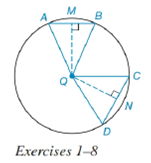Chapter 6.4, Problem 7EElementary Geometry For College St...

7th Edition
Alexander + 2 others
ISBN: 9781337614085

Solutions

Chapter
SectionElementary Geometry For College St...

7th Edition
Alexander + 2 others
ISBN: 9781337614085
Textbook Problem

In Exercises 1 to 8, use the figure provided.If m C D ⌢ : m A B ⌢ = 3 : 2 , write an inequality that compares Q M to Q N .To determine

To write:

An inequality that compares QM to QN.

Explanation

Given:

mCD:mAB=3:2 and the figure given below

Theorem:

In a circle (or in congruent circles) containing two unequal chords, the shorter chord is at the greater distance from the center of the circle.

In a circle (or in congruent circles) containing two unequal minor arcs, the greater minor arc corresponds to the longer of the chords related to these arcs.

Calculation:

Since mCD:mAB=3:2, it means that mCD<mAB.

Using the theorem.

And since the arcs are unequal i.e. mCD<mAB and from the given figure

Still sussing out bartleby?

Check out a sample textbook solution.

See a sample solution

The Solution to Your Study Problems

Bartleby provides explanations to thousands of textbook problems written by our experts, many with advanced degrees!

Get Started

Calculate y'. 34. y = 10tan

Calculus: Early Transcendentals

Express the limit as a derivative and evaluate. 108. lim/3cos0.5/3

Single Variable Calculus: Early Transcendentals, Volume I

Fill in each blank: 14qt=gal

Elementary Technical Mathematics

True or False: The graph in question 3 has a vertical asymptote at x = 1.

Study Guide for Stewart's Single Variable Calculus: Early Transcendentals, 8th